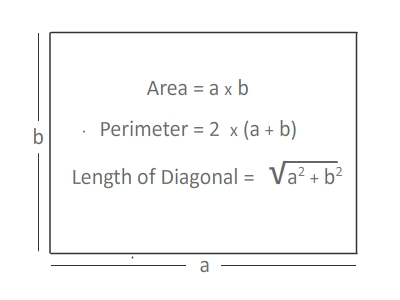# Rectangle Area, Perimeter and Length of Diagonal Calculator

Rectangle Area Calculator tool is used to calculate area, perimeter and length of the diagonal of a rectangle; given side 1 and side 2 by user.

## What is Rectangle?

A rectangle is a 2D shape in, having 4 sides and 4 corners. Its two meet at . Thus, a rectangle has 4 , each measuring 90 ̊. The opposite sides of a rectangle have the same lengths and are parallel. Two sides are said to be parallel, when the distance between them remains the same at all points.

Area of Rectangle

### Rectangle Formula

Area of rectangle is the product of Length and Width; Area A = L X W or A = a x b.

Perimeter of Rectangle

The perimeter of a rectangle is the sum of its four sides. Since a rectangle has two equal short sides (width, b) and two equal long sides (length, a), perimeter=2a + 2b

Length of the Diagonal

A diagonal of a rectangle cuts the rectangle into two right triangles with sides equal to the sides of the rectangle and with a hypotenuse that is the diagonal. By using Pythagorous theorem, we can easily find the length of the rectangle.

Length of the Diagonal=sqrt(Length2 + Width2)

#### Fun Facts About Rectangle in Geometry

• Opposite sides are equal and parallel.
• All angles are equal to 90 degrees.
• Opposite sides are equal and parallel.
• All angles are equal to 90 degrees.
• The diagonals are equal and bisect each other.
• The intersection of the diagonals is the circumcentre. That is you can draw a circle with that as centre to pass through the four corners.
• Lines joining the mid points of the sides of a rectangle in an order form a rhombus of half the area of the rectangle.
• The sum of the four exterior angles is 4 right angles.
• The sum of the four interior angles is 4 right angles.
• Join the opposite sides of a rectangle and you get four congruent rectangles of one-fourth the size of the main rectangle.
• Join the midpoints of the sides in order and you get a parallelogram whose sides are parallel to the diagonals.### Spreading Knowledge Across the World

USA - United States of America  Canada  United Kingdom  Australia  New Zealand  South America  Brazil  Portugal  Netherland  South Africa  Ethiopia  Zambia  Singapore  Malaysia  India  China  UAE - Saudi Arabia  Qatar  Oman  Kuwait  Bahrain  Dubai  Israil  England  Scotland  Norway  Ireland  Denmark  France  Spain  Poland  and  many more....